# Fraction calculator

The calculator performs basic and advanced operations with fractions, expressions with fractions combined with integers, decimals, and mixed numbers. It also shows detailed step-by-step information about the fraction calculation procedure. Solve problems with two, three, or more fractions and numbers in one expression.

## Result:

### 3 / 1/2 = 6/1 = 6

Spelled result in words is six.

### How do you solve fractions step by step?

1. Divide: 3 : 1/2 = 3/1 · 2/1 = 3 · 2/1 · 1 = 6/1 = 6
Dividing two fractions is the same as multiplying the first fraction by the reciprocal value of the second fraction. The first sub-step is to find the reciprocal (reverse the numerator and denominator, reciprocal of 1/2 is 2/1) of the second fraction. Next, multiply the two numerators. Then, multiply the two denominators.
In words - three divided by one half = six.

#### Rules for expressions with fractions:

Fractions - use the slash “/” between the numerator and denominator, i.e., for five-hundredths, enter 5/100. If you are using mixed numbers, be sure to leave a single space between the whole and fraction part.
The slash separates the numerator (number above a fraction line) and denominator (number below).

Mixed numerals (mixed fractions or mixed numbers) write as non-zero integer separated by one space and fraction i.e., 1 2/3 (having the same sign). An example of a negative mixed fraction: -5 1/2.
Because slash is both signs for fraction line and division, we recommended use colon (:) as the operator of division fractions i.e., 1/2 : 3.

Decimals (decimal numbers) enter with a decimal point . and they are automatically converted to fractions - i.e. 1.45.

The colon : and slash / is the symbol of division. Can be used to divide mixed numbers 1 2/3 : 4 3/8 or can be used for write complex fractions i.e. 1/2 : 1/3.
An asterisk * or × is the symbol for multiplication.
Plus + is addition, minus sign - is subtraction and ()[] is mathematical parentheses.
The exponentiation/power symbol is ^ - for example: (7/8-4/5)^2 = (7/8-4/5)2

#### Examples:

subtracting fractions: 2/3 - 1/2
multiplying fractions: 7/8 * 3/9
dividing Fractions: 1/2 : 3/4
exponentiation of fraction: 3/5^3
fractional exponents: 16 ^ 1/2
adding fractions and mixed numbers: 8/5 + 6 2/7
dividing integer and fraction: 5 ÷ 1/2
complex fractions: 5/8 : 2 2/3
decimal to fraction: 0.625
Fraction to Decimal: 1/4
Fraction to Percent: 1/8 %
comparing fractions: 1/4 2/3
multiplying a fraction by a whole number: 6 * 3/4
square root of a fraction: sqrt(1/16)
reducing or simplifying the fraction (simplification) - dividing the numerator and denominator of a fraction by the same non-zero number - equivalent fraction: 4/22
expression with brackets: 1/3 * (1/2 - 3 3/8)
compound fraction: 3/4 of 5/7
fractions multiple: 2/3 of 3/5
divide to find the quotient: 3/5 ÷ 2/3

The calculator follows well-known rules for order of operations. The most common mnemonics for remembering this order of operations are:
PEMDAS - Parentheses, Exponents, Multiplication, Division, Addition, Subtraction.
BEDMAS - Brackets, Exponents, Division, Multiplication, Addition, Subtraction
BODMAS - Brackets, Of or Order, Division, Multiplication, Addition, Subtraction.
GEMDAS - Grouping Symbols - brackets (){}, Exponents, Multiplication, Division, Addition, Subtraction.
Be careful, always do multiplication and division before addition and subtraction. Some operators (+ and -) and (* and /) has the same priority and then must evaluate from left to right.

## Fractions in word problems:

• Cups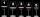We have three cups. In the cups we had fluid and boredom we started to shed. 1 We shed one-third of the fluid from the second glass into the first and third. 2 Then we shed one quarter cup of liquid from the first to the second and to the third. 3 Then we
• DayWhat part of the day are 23 hours 22 minutes? Express as a decimal number.
• University bubbleYou'll notice that the college up slowly every other high school. In Slovakia/Czech republic a lot of people studying political science, mass media communication, social work, many sorts of management MBA. Calculate how many times more earns clever 25-yea
• Gear wheelsTwo gear wheels, which fit together, have the number of teeth z1=58 and z2=149. Calculate the speed of the first wheel, if the second wheel rotates 1232 revolutions per minute.
• Camp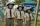In the camp are children. 1/2 went on a trip, 1/4 went to bathe, and 38 children remained in the room. How many children are in the camp?
• Equation - inverseSolve for x: 7: x = 14: 1000
• Julian 2Julian and two of his friends are going to share 1/4 of a pizza. How much will each person get?
• Quotient and divisionWhat is the quotient of 2/9 and 1/8?
• Reciprocals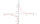Which among the given reciprocal is correct a. 3/15x1/3= 1 b. 3/20x20/3=1 c. 7/14x7/7=1 d. 34/3x34/34=1
• AlmondsRudi has 4 cups of almonds. His trail mix recipe calls for 2/3 cup of almonds. How many batches of trail mix can he make?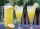Six friends are sharing 8 cups of lemonade. If they share the lemonade equally, how many cups will each friend get?Lauren is tutoring students at the library on Saturday. If she is at the library for a total of 6 hours and she helps each student, one at a time, for three-fourths of an hour, how many students does she tutor?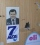A landscaper has 1/5 ton of dirt. She distributes it equally across three plots of land. What fraction of the dirt does each plot get?